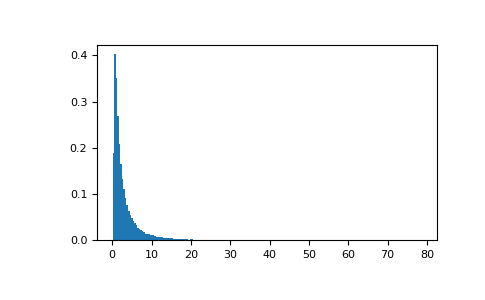# numpy.random.mtrand.RandomState.wald¶

method

RandomState.wald(mean, scale, size=None)

Draw samples from a Wald, or inverse Gaussian, distribution.

As the scale approaches infinity, the distribution becomes more like a Gaussian. Some references claim that the Wald is an inverse Gaussian with mean equal to 1, but this is by no means universal.

The inverse Gaussian distribution was first studied in relationship to Brownian motion. In 1956 M.C.K. Tweedie used the name inverse Gaussian because there is an inverse relationship between the time to cover a unit distance and distance covered in unit time.

Parameters: mean : float or array_like of floats Distribution mean, must be > 0. scale : float or array_like of floats Scale parameter, must be > 0. size : int or tuple of ints, optional Output shape. If the given shape is, e.g., (m, n, k), then m * n * k samples are drawn. If size is None (default), a single value is returned if mean and scale are both scalars. Otherwise, np.broadcast(mean, scale).size samples are drawn. out : ndarray or scalar Drawn samples from the parameterized Wald distribution.

Notes

The probability density function for the Wald distribution isAs noted above the inverse Gaussian distribution first arise from attempts to model Brownian motion. It is also a competitor to the Weibull for use in reliability modeling and modeling stock returns and interest rate processes.

References

  Brighton Webs Ltd., Wald Distribution, https://web.archive.org/web/20090423014010/http://www.brighton-webs.co.uk:80/distributions/wald.asp
  Chhikara, Raj S., and Folks, J. Leroy, “The Inverse Gaussian Distribution: Theory : Methodology, and Applications”, CRC Press, 1988.
  Wikipedia, “Inverse Gaussian distribution” https://en.wikipedia.org/wiki/Inverse_Gaussian_distribution

Examples

Draw values from the distribution and plot the histogram:

>>> import matplotlib.pyplot as plt
>>> h = plt.hist(np.random.wald(3, 2, 100000), bins=200, density=True)
>>> plt.show()#### Previous topic

numpy.random.mtrand.RandomState.vonmises

#### Next topic

numpy.random.mtrand.RandomState.weibull